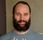cancel
Showing results for
Did you mean:Frequent Visitor

## [SSAS] Measure := IF [column1] = "ABCD" then DIVIDE()... else... DIVIDE()

Hey everyone,

I have a IF condition that I need to replicate in DAX measure. It looks like that:

Measure := IF [column1] = 'Y' then DIVIDE(sum([col2),sum(col2)+sum(col3)) else DIVIDE(sum([col2]),sum[col2]).

Else condition of course can be hardcoded as "1" but that's not a main issue here.

As we know, DAX measures can't use IFs based on Columns in the model/data so what would be the best way of implementing this in DAX measure(!) [this is important as I'm working with SSAS not PBI]?

3 REPLIES 3Super User

If you have column1 on axis or slicers you can do this:
measure = if(selectedvalue(Table[column1])="Y";......

If  you want to have the sum across all values in column, but with different calculations for them you can try this
measure = sumx(table,if(selectedvalue(Table[column1])=1;....)Frequent Visitor

@sturlaws, thanks. Column1 is more of used as flag column and is not used later in the report/filters/is vislble to users at all.

So not sure whether selectedvalue() will be proper here.Super User

The selectedvalue() has perhaps a bit misleading name, but these two expressions are  equivalent

`SELECTEDVALUE( <columnName>, <alternateResult>)`
`IF(HASONEVALUE(<columnName>), VALUES(<columnName>), <alternateResult>)`

If the filter context has narrowed the values in the column down one unique value, this value is returned, otherwise a blank or alternative value is returned: https://dax.guide/selectedvalue/

If column 1 is a flag value, and not something you will add in a report the SUMX-version is probably what will give you what you need.